# Test: Steel Design- 2

## 20 Questions MCQ Test Mock Test Series of SSC JE Civil Engineering | Test: Steel Design- 2

Description
Attempt Test: Steel Design- 2 | 20 questions in 12 minutes | Mock test for SSC preparation | Free important questions MCQ to study Mock Test Series of SSC JE Civil Engineering for SSC Exam | Download free PDF with solutions
QUESTION: 1

### The effective length of a fillet weld should not be less than

Solution:

The effective length of the weld is the length of the weld for which specified size and throat thickness exist. In drawings only effective length is shown, while welding length made equal to effective length plus twice the size of the weld.

Effective length should not be less than 4 times the size of weld.

QUESTION: 2

### According to the Unwin’s formula, if t is the thickness of the plate in mm, the nominal diameter of the rivet is

Solution:

According to Unwin’s formula,

The nominal diameter (ɸ) of rivet is given by:

ɸ = 6.01√t

where,

t = thickness of plate in “mm”

QUESTION: 3

### Pick up the correct statement from the following:

Solution:
QUESTION: 4

According to IS 800-1984, the minimum thickness of vertically stiffened web plate shall not be less than:

Solution:

As per I.S: 800-1984, the thickness of the web plate should not be less than the following as appropriate: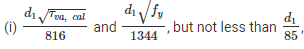for unstiffened web.

(ii) 1/180 of smallest clear panel dimension and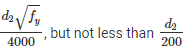for vertically stiffened web.

(iii) 1/180 of the smaller dimension in each panel and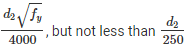for webs stiffened both vertically and horizontally with a horizontal stiffener at a distance from the compression flange equal to 2/5th of the distance from the compression flange to the neutral axis.

(iv) 1/180 smaller clear panel dimension and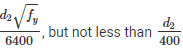when there is also a horizontal stiffener at the neutral axis, where d2 is twice the clear distance from the compression flange angles, or plate or tongue plate to the neutral axis.

QUESTION: 5

Design of riveted joint is based on the assumption:

Solution:

Followings are the assumptions in the design of riveted connections:

i) Load is assumed to be uniformly distributed among all the rivets.

ii) Stress in plate is assumed to be uniform.

iii) Shear stress is assumed to be uniformly distributed over the gross area of rivets.

iv) Bearing stress is assumed to be uniform between the contact surfaces of plate and rivet.

v) Bending stress in rivet is neglected.

vi) Rivet hole is assumed to be completely filled by the rivet.

vii) Friction between plates is neglected.

QUESTION: 6

The best arrangement to provide unified behaviour in built up steel column is by:

Solution:

Two rolled steel sections are connected to each other by lacing, battening, tie plates etc. Lacings (and even battens) are not the load carrying members, they are basically provided so that two columns which they attach work as single entity.

i) Lacing is generally preferred in case of eccentric loads. Flat bars are generally used for lacing. Angles, channels and tubular sections are also used for lacing of very heavily columns.

ii) Battening is normally used for axially loaded columns and in sections where the components are not far apart. Plates are used for battens.

QUESTION: 7

The internal pressure coefficient on walls for buildings with large permeability is taken as:

Solution:

According to IS 875: Part 3

Buildings with medium and large openings - Buildings with medium and large openings may also exhibit either positive or negative internal pressure depending upon the direction of wind.

Buildings with medium openings between about 5 to 20 percent of wall area shall be examined for an internal pressure coefficient of +0.5 and later with an internal pressure coefficient of -0.5, and the analysis which produces greater distress of the members shall be adopted.

Buildings with large openings, that is, openings larger than 20 percent of the wall area shall be examined once with an internal pressure coefficient of -0.7 and again with an internal pressure coefficient of -0.7, and the analysis which produces greater distress on the members shall be adopted.

QUESTION: 8

If the loaded length of span in meters of a railway steel bridge carrying a single track is 6 m, then impact factor is taken as:

Solution:

Impact factor is given by: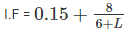Where,

L = span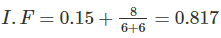i.e, Impact factor lies between 0.5 and 1.0

QUESTION: 9

If “Ib” is the moment of the inertia of the rolled beam section, “Ap” is the area of the cover plates in one flange and “h” is the distance between the centroid of the top and bottom flange plates, Moment of inertia of Built-up plate girder is given by:

Solution:

Moment of inertia of Built-up plate girder is given by: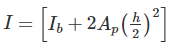Where,

I= is the moment of the inertia of the rolled beam section

A= is the area of the cover plates in one flange

h = is the distance between the centroid of the top and bottom flange plates

QUESTION: 10

The fillet weld whose axis is perpendicular to the direction of the applied load is known as:

Solution:
QUESTION: 11

The beam sections in which the extreme fibre in compression can yield stress, but cannot develop the plastic moment of resistance, due to local buckling are classified as

Solution:
QUESTION: 12

If fillet weld is applied to the rounded toe of a rolled steel section having thickness of "t" at toe, the specified size of weld generally not to exceed

Solution:

Fillet weld is provided to square edges, the weld size should be at least 1.5 mm less than the edge thickness (i.e Thickness of thinner member – 1.5 mm)

For the rounded toe of a rolled section, the weld size should not exceed ¾ thickness of the section at the toe (i.e = 3/4th thickness of rolled section at toe)

QUESTION: 13

If a plate with small hole is kept over another plate and the entire hole is filled with weld material, the welded joint is known as

Solution:
QUESTION: 14

A simply supported beam of rectangular section and span L is subjected to a concentrated load at the mid span. The length of the plastic hinge is–

Solution:
QUESTION: 15

Consider the following with respect to lacing for steel column:

1. It is required to resist a total transverse shear of 2.5 percent of the axial and bending stress.

2. The slenderness ratio of lacing bars shall not exceed 135.

3. For single lacing, effective length is the distance between the inner rivets of the bars.

4. For double lacing, effective length is the distance between the outer most rivets of the bars.

Which one of the above statements are correct?

Solution:

(i) Total transverse shear (V) = 2.5% axial load.

(ii) Slenderness ration of lacing bars ≯ 145

(iii) For both single lacing and double lacing, effective length of lacing is taken as the distance between inner rivets.

QUESTION: 16

In case of tension member consisting of two angles back to back of same side of gusset plate, and members are tack riveted. what is K equal to?

Where, A1 – Area of connected leg

A2 – Area of outstanding leg

Solution:
QUESTION: 17

If a rolled steel flat designated as 55 I.S.F. 12 mm is used as lacing, then minimum radius of gyration will be ________.

Solution:

The minimum radius of gyration,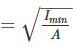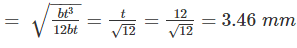QUESTION: 18

When two plates are placed end to end and are joined by two cover plates, the joint is known as _____.

Solution:
QUESTION: 19

According to IS 800 – 2007, the permissible bending stress in column base in case of Fe415 is:

Solution:

As per IS 800:2007, clause 11.3.3 the permissible bending stress in column base is 0.75 fy.

So, permissible bending stress is 0.75 x 415= 311 MPa

QUESTION: 20

The critical section for web crippling in case of flexure members is at:

Solution:

High concentrated loads create large bearing stress concentration at the junction of web and flange. The web near the portion of stress concentration tends to fold over the flange. This type of local buckling phenomenon is called as web crippling. The rot of the fillet weld is critical section for web crippling.Use Code STAYHOME200 and get INR 200 additional OFF Use Coupon Code